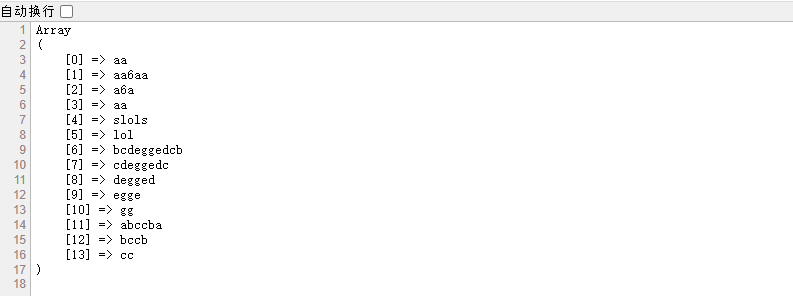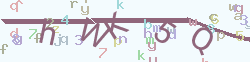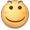•微信

# php设计函数getMaxStr，找出一个字符串中的最长对称字符串-[原创]

```\$str='aa6aaslolsbcdeggedcbaiokabccbanh';

function getMaxStr(\$str){
\$s_data=str_split(\$str);
\$row=[];
foreach(\$s_data as \$key=>\$vol){
foreach(\$s_data as \$k=>\$v){
if(\$k<\$key) continue;
if(\$vol==\$v && \$key!=\$k){
if((\$key+\$k)==1){
\$row[]=substr(\$str,\$key,2);
}else{
\$px=\$key==0?\$k+1:\$k-\$key;
\$px=(\$px%2)==0?\$px:(\$k-\$key+1);
\$px=\$px/2;
for(\$s=1;\$s<\$px;\$s++){
if(\$s_data[\$key+\$s]!=\$s_data[\$k-\$s]) continue 2;
}
\$row[]=substr(\$str,\$key,(\$k-\$key+1));
}
}
}
}
return \$row;
}

\$r=getMaxStr(\$str);
print_r(\$r);```